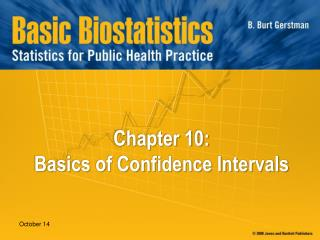DownloadDownload PresentationChapter 10: Basics of Confidence Intervals

# Chapter 10: Basics of Confidence Intervals

Download Presentation## Chapter 10: Basics of Confidence Intervals

- - - - - - - - - - - - - - - - - - - - - - - - - - - E N D - - - - - - - - - - - - - - - - - - - - - - - - - - -
##### Presentation Transcript

1. Chapter 10: Basics of Confidence Intervals

2. In Chapter 10: 10.1 Introduction to Estimation 10.2 Confidence Interval for μ when σ is known 10.3 Sample Size Requirements 10.4 Relationship Between Hypothesis Testing and Confidence Intervals

3. §10.1: Introduction to Estimation Two forms of estimation • Point estimation ≡ single best estimate of parameter (e.g., x-bar is the point estimate of μ) • Interval estimation ≡ surrounding the point estimate with a margin of error to create a range of values that seeks to capture the parameter; a confidence interval

4. Reasoning Behind a 95% Confidence Interval • A schematic (next slide) of a sampling distribution of means based on repeated independent SRSs of n = 712 is taken from a population with unknown μ and σ = 40. • Each sample derives a different point estimate and 95% confidence interval • 95% of the confidence intervals will capture the value of μ

5. Confidence Intervals • To create a 95% confidence interval for μ, surround each sample mean with a margin of error m that is equal to 2standard errors of the mean:m ≈ 2×SE = 2×(σ/√n) • The 95% confidence interval for μ is now

6. This figure shows a sampling distribution of means. Below the sampling distribution are five confidence intervals. In this instance, all but the third confidence captured μ

7. Example: Rough Confidence Interval Suppose body weights of 20-29-year-old males has unknown μ and σ = 40. I take an SRS of n = 712 from this population and calculate x-bar =183. Thus:

8. Confidence Interval Formula Here is a better formula for a (1−α)100% confidence interval for μ when σ is known: Note that σ/√n is the SE of the mean

9. Common Levels of Confidence

10. 90% Confidence Interval for μ Data: SRS, n = 712, σ = 40, x-bar = 183

11. 95% Confidence Interval for μ Data: SRS, n = 712, σ = 40, x-bar = 183

12. 99% Confidence Interval for μ Data: SRS, n = 712, σ = 40, x-bar = 183

13. Confidence Level and CI Length ↑ confidence costs  ↑ confidence interval length

14. 10.3 Sample Size Requirements To derive a confidence interval for μ with margin of error m, study this many individuals:

15. Examples: Sample Size Requirements Suppose we have a variable with s= 15 and want a 95% confidence interval. Note, α = .05  z1–.05/2 = z.975= 1.96 round up to ensure precision Smaller margins of error require larger sample sizes

16. 10.4 Relationship Between Hypothesis Testing and Confidence Intervals A two-sided test will reject the null hypothesis at the α level of significance when the value of μ0 falls outside the (1−α)100% confidence interval This illustration rejects H0: μ = 180 at α =.05 because 180 falls outside the 95% confidence interval. It retains H0: μ = 180 at α = .01 because the 99% confidence interval captures 180.